# Which of the following fatty acids would you expect to have the lowest melting point? Which...

Which of the following fatty acids would you expect to have the lowest melting point?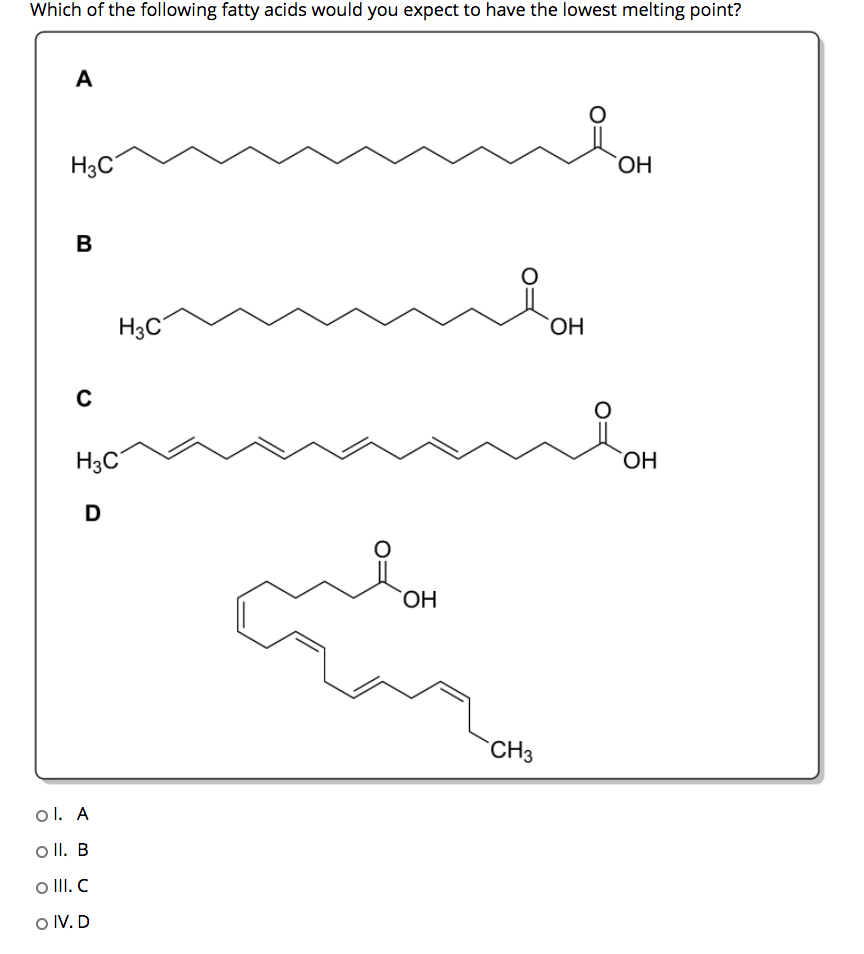Which of the following fatty acids would you expect to have the lowest melting point? A Нас ОН В НЫС ОН C H3C ОН D ОН CH3 ol. A o II. B o III.C o IV. D

Lesser the surface area means more the circular structure, lesser will be the intermolecular force. Lesser the intermolecular force lesser will be the melting point. So, here option-D is the answer.

##### Add Answer of: Which of the following fatty acids would you expect to have the lowest melting point? Which...
Similar Homework Help Questions
• ### Which of the following fatty acids would you expect to have the lowest melting point? Which...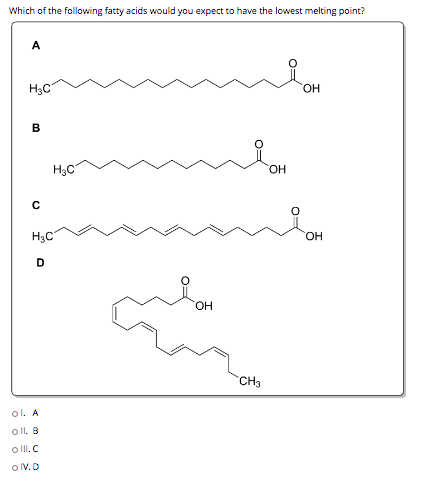Which of the following fatty acids would you expect to have the lowest melting point? Which of the following fatty acids would you expect to have the lowest melting point? A ныс ОН B Р H3C ОН с о H3C ОН D ОН CH3 ol. A Oll. В olll. IV.D

• ### Which of the following fatty acids would you expect to have the lowest melting point? A...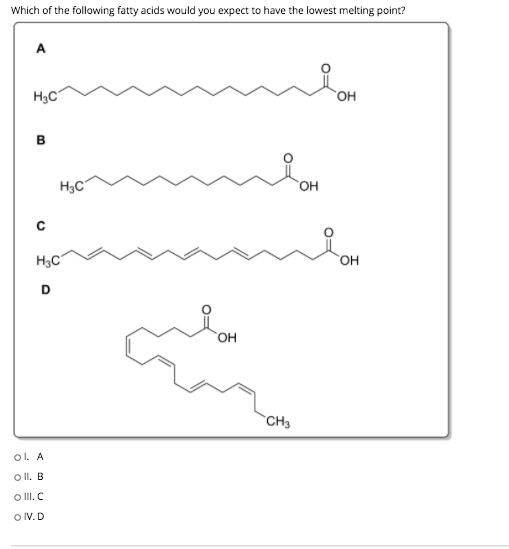Which of the following fatty acids would you expect to have the lowest melting point? A нс ОН B нэс он с нэс он D он сн. ol. A o II, В o 11. С O IV.D

• ### QUESTION 23 Which of the following fatty acids would you expect to have the lowest melting...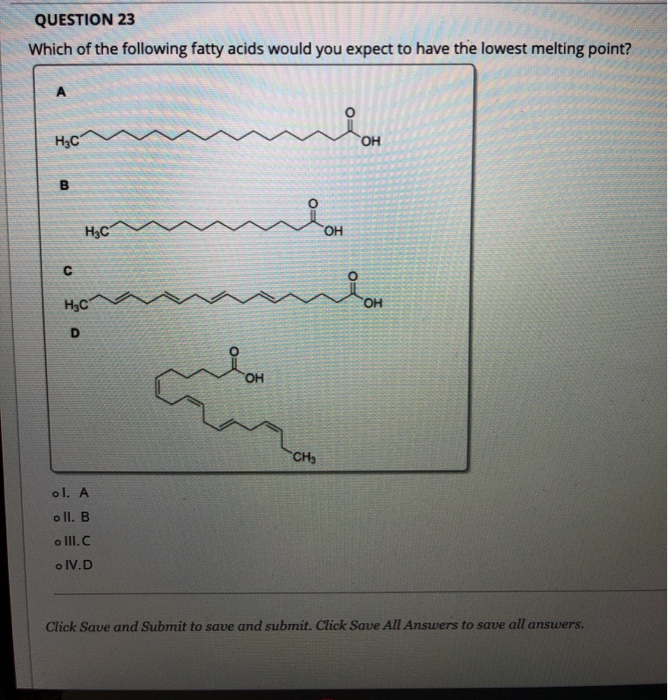QUESTION 23 Which of the following fatty acids would you expect to have the lowest melting point? А O= нс OH B H₃C' OH C нус OH D OH CH3 ol. A oll. B o III.C .IV.D Click Save and Submit to save and submit. Click Save All Answers to save all answers.

• ### #1 please answer all components. Question 1 (6 points) Consider the following fatty acids. Label each one as saturated, monounsaturated, polyunsaturated, or trans. Circle the one you would expect...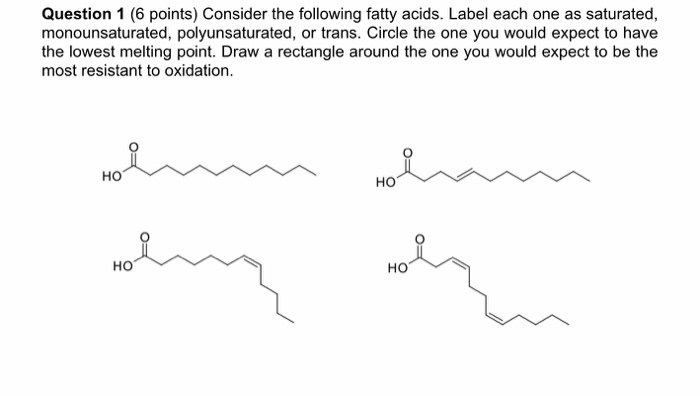#1 please answer all components. Question 1 (6 points) Consider the following fatty acids. Label each one as saturated, monounsaturated, polyunsaturated, or trans. Circle the one you would expect to have the lowest melting point. Draw a rectangle around the one you would expect to be the most resistant to oxidation но но но HO Question 1 (6 points) Consider the following fatty acids. Label each one as saturated, monounsaturated, polyunsaturated, or trans. Circle the one you would expect to...

• ### Select the major product of the following reaction scheme: Select the major product of the following...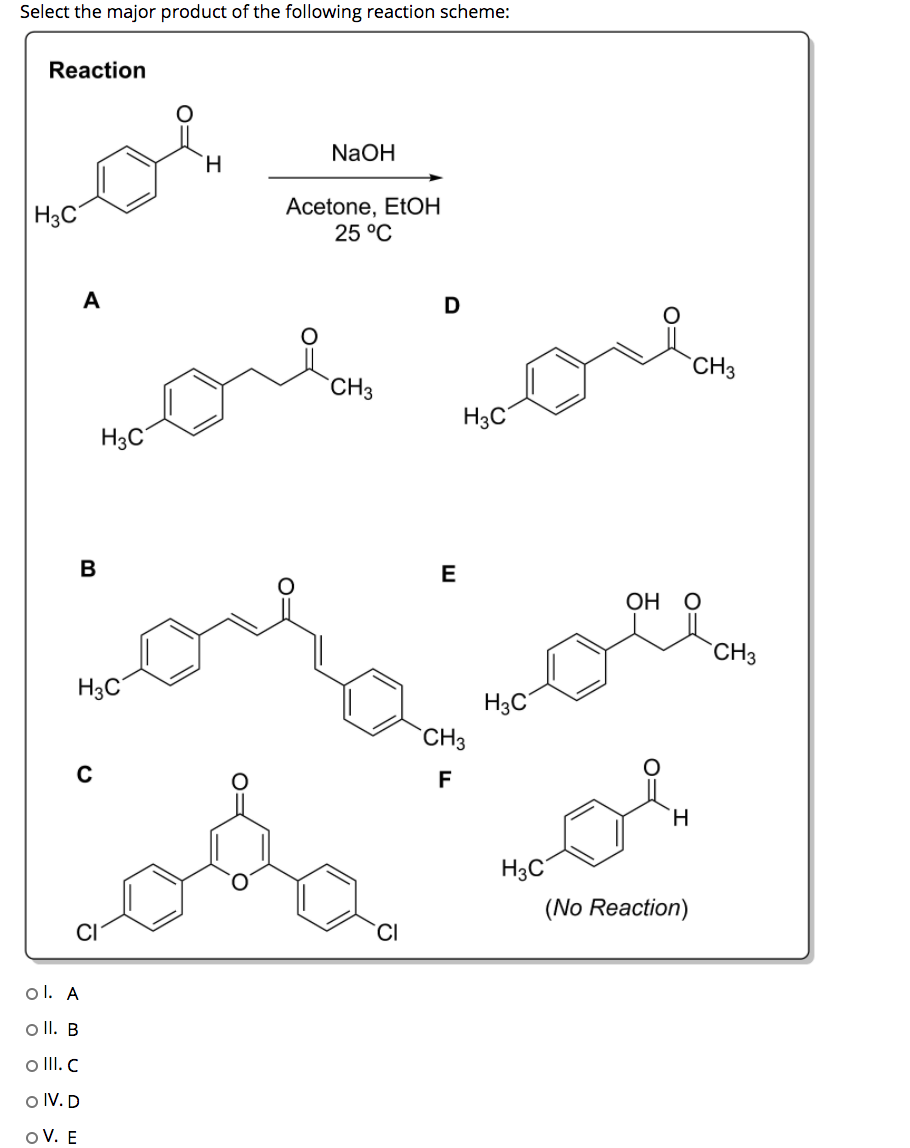Select the major product of the following reaction scheme: Select the major product of the following reaction scheme: Reaction NaOH H H3C Acetone, EtOH 25 °C А D CH3 CH3 H3C H3C B E ОН О CH3 H3C H3C CH3 C F H H3C (No Reaction) CI ol. A o II. B o III.C o IV.D OV. E

• ### which of the following would you expect to have a longer lambda max? explain? pls answer all the questions Which of...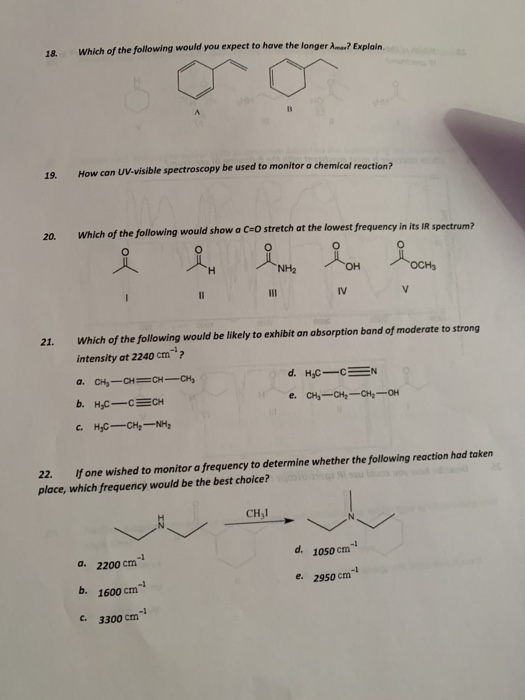which of the following would you expect to have a longer lambda max? explain? pls answer all the questions Which of the following would you expect to have the longer Ama? Explain. 18. How can UV-visible spectroscopy be used to monitor a chemical reaction? 19. Which of the following would show a C-O stretch at the lowest frequency in its IR spectrum? 20. O NH2 ОН OCH3 I1 II IV V Which of the following would be likely to exhibit...

• ### 1. List the fatty acids below (by their letter) in order from lowest melting point to highest. OH OH c) CH,– (CH), -...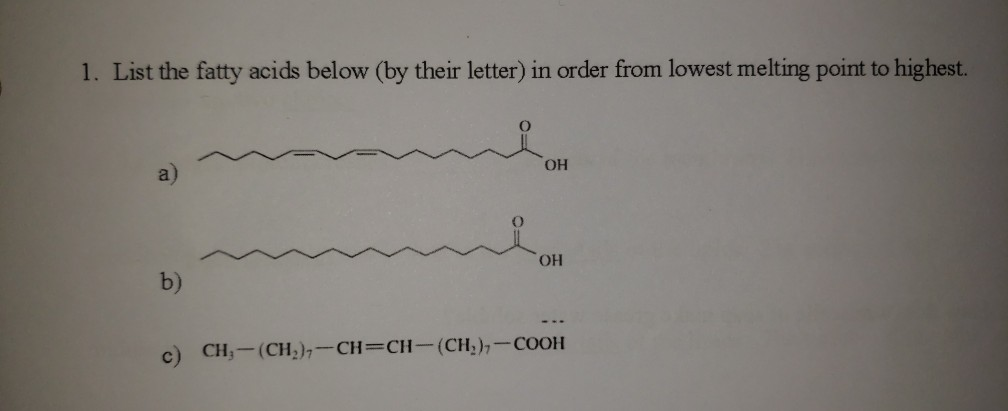1. List the fatty acids below (by their letter) in order from lowest melting point to highest. OH OH c) CH,– (CH), -CH=CH-(CHR)--COOH

• ### 4. A list of fatty acids is given below. The number of carbons each contains, as...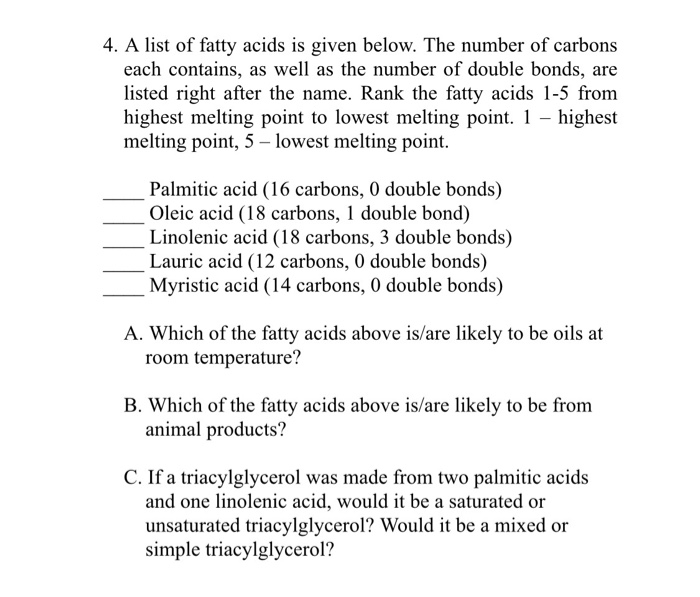4. A list of fatty acids is given below. The number of carbons each contains, as well as the number of double bonds, are listed right after the name. Rank the fatty acids 1-5 from highest melting point to lowest melting point. 1 - highest melting point, 5 - lowest melting point. Palmitic acid (16 carbons, 0 double bonds) Oleic acid (18 carbons, 1 double bond) Linolenic acid (18 carbons, 3 double bonds) Lauric acid (12 carbons, 0 double bonds)...

• ### of the five labeled protons in this molecule, which do you expect would have the highest...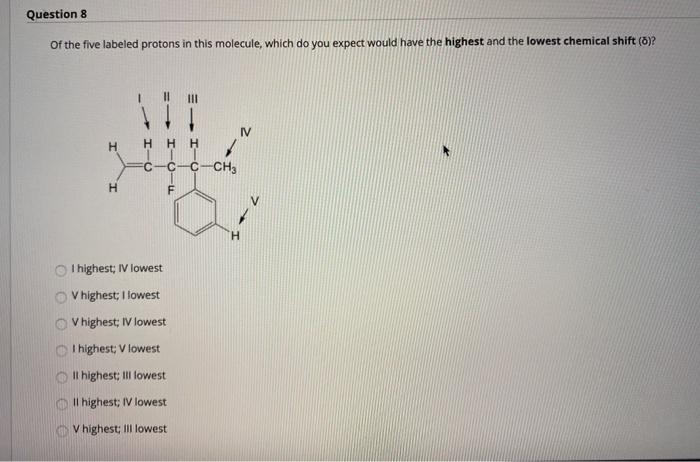of the five labeled protons in this molecule, which do you expect would have the highest and the lowest chemical shift? Question 8 Of the five labeled protons in this molecule, which do you expect would have the highest and the lowest chemical shift (8)? U III H Η Η Η C C-C-CH3 H F H I highest; IV lowest V highest; I lowest V highest; IV lowest I highest; V lowest II highest; III lowest Oll highest; IV lowest...

• ### Which of the following pairs of fatty acids would have the higher melting temperature? 1) C18:0...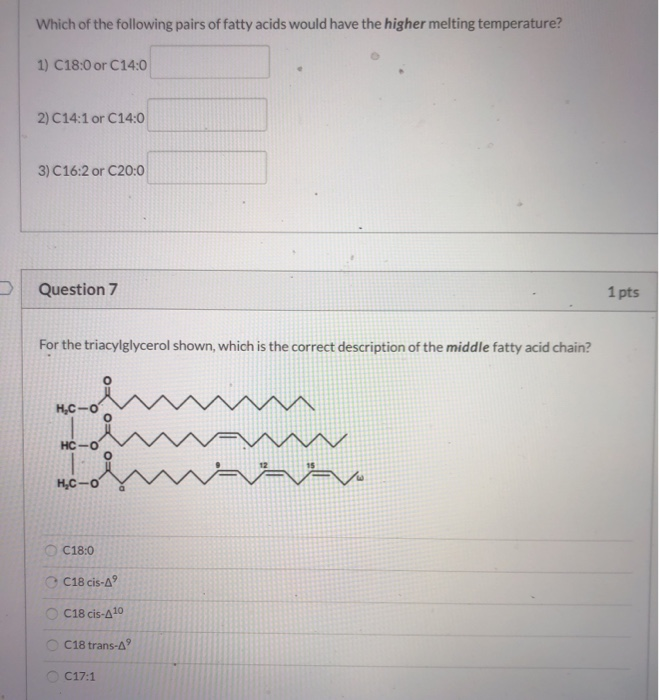Which of the following pairs of fatty acids would have the higher melting temperature? 1) C18:0 or C14:0 2) C14:1 or C14:0 3) C16:2 or C20:0 Question 7 1 pts For the triacylglycerol shown, which is the correct description of the middle fatty acid chain? H.C-00 HC-0 H.C-0 ー C18:0 C18 cis-A C18 cis-410 C18 trans- C17:1

Free Homework App